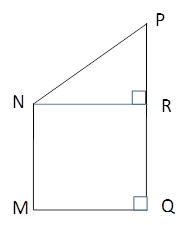Chapter 4.4, Problem 44EElementary Geometry For College St...

7th Edition
Alexander + 2 others
ISBN: 9781337614085

Solutions

Chapter
SectionElementary Geometry For College St...

7th Edition
Alexander + 2 others
ISBN: 9781337614085
Textbook Problem

With M N ¯ | | Q P ¯ and ∠ M ≅ ∠ Q , MNPQ is a right trapezoid. Finda) m ∠ P , if m ∠ M N P − m ∠ P = 54 ° b) the length of the side N P ¯ , if MN = 15 cm, MQ = 12 cm, and PQ = 20 cm.Exercises 43, 44

To determine

(a)

To Find:

mP

Explanation

Consider the following diagram.

Given: A right trapezoid MNPQ with MN¯||QP¯ and MQ.

Also, mMNPmP=54°.

Here, MN¯||QP¯ and MQ.

Therefore, mM=mQ=90°.

It is given that mMNPmP=54°

To determine

(b)

To Find:

The length of NP¯.

Still sussing out bartleby?

Check out a sample textbook solution.

See a sample solution

The Solution to Your Study Problems

Bartleby provides explanations to thousands of textbook problems written by our experts, many with advanced degrees!

Get Started

Compute C7,7.

Understanding Basic Statistics

Given f(x)=x2+3, evaluate each expression. f(x+x) b. f(x+x)f(x)x

Calculus: An Applied Approach (MindTap Course List)

Evaluate the integrals in Problems 7-36. Check your results by differentiation. 15.

Mathematical Applications for the Management, Life, and Social Sciences

Use the guidelines of this section to sketch the curve. y=x2+xx

Single Variable Calculus: Early Transcendentals, Volume I

Differentiate. y = sec tan

Calculus: Early Transcendentals

True or False: is a convergent series.

Study Guide for Stewart's Single Variable Calculus: Early Transcendentals, 8th